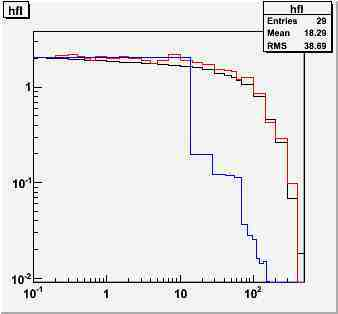# GetRandom numbers according to TH1D (variable bin size)

Hello,

I am trying to produce random numbers according to the data of a spectrum with bins of variable size. I use: h->GetRandom() to generate energies following this spectrum. Then I fill an output histogram with the randomly generated numbers.

The problem is that, depending on how I define the bins of the histogram that I fill, the result looks different (see attached picture):

• If the histogram is defined with the same bins as the original one, the result looks good (red line).
• If I define the histogram with a constant bin size, I see the steps of the original bins (blue line).

I would like that the data is smoothly distributed, following the original smooth line, and without depending on how the output histogram is defined. Is it possible to do that?

I send also attached a data file and a function to reproduce the problem, if needed.

Best regards,
Estela.
test.C (1.99 KB)
data.txt (836 Bytes)To compare plots with different bin widths, you should divide your histograms bin by bin by the bin width

Rene